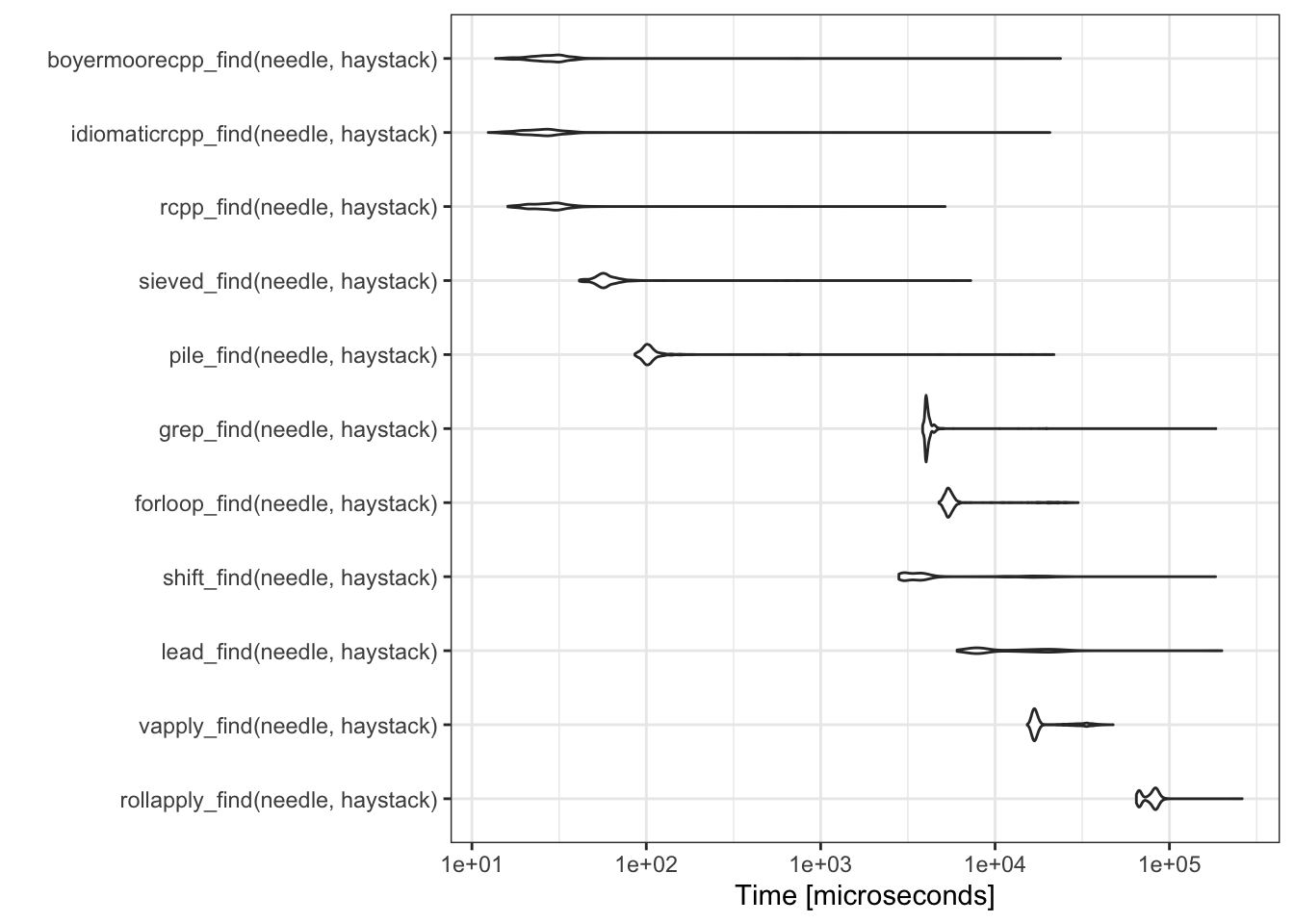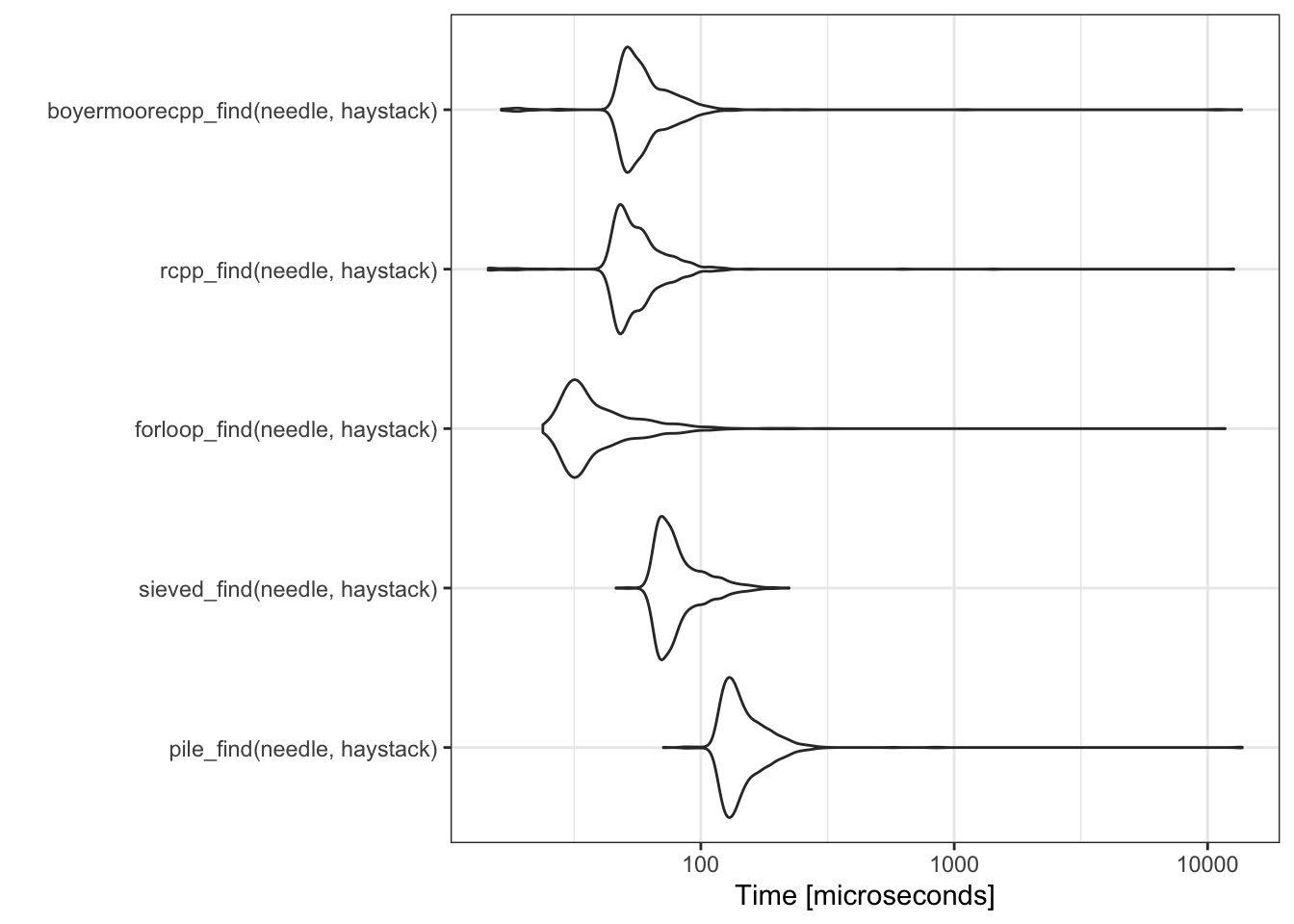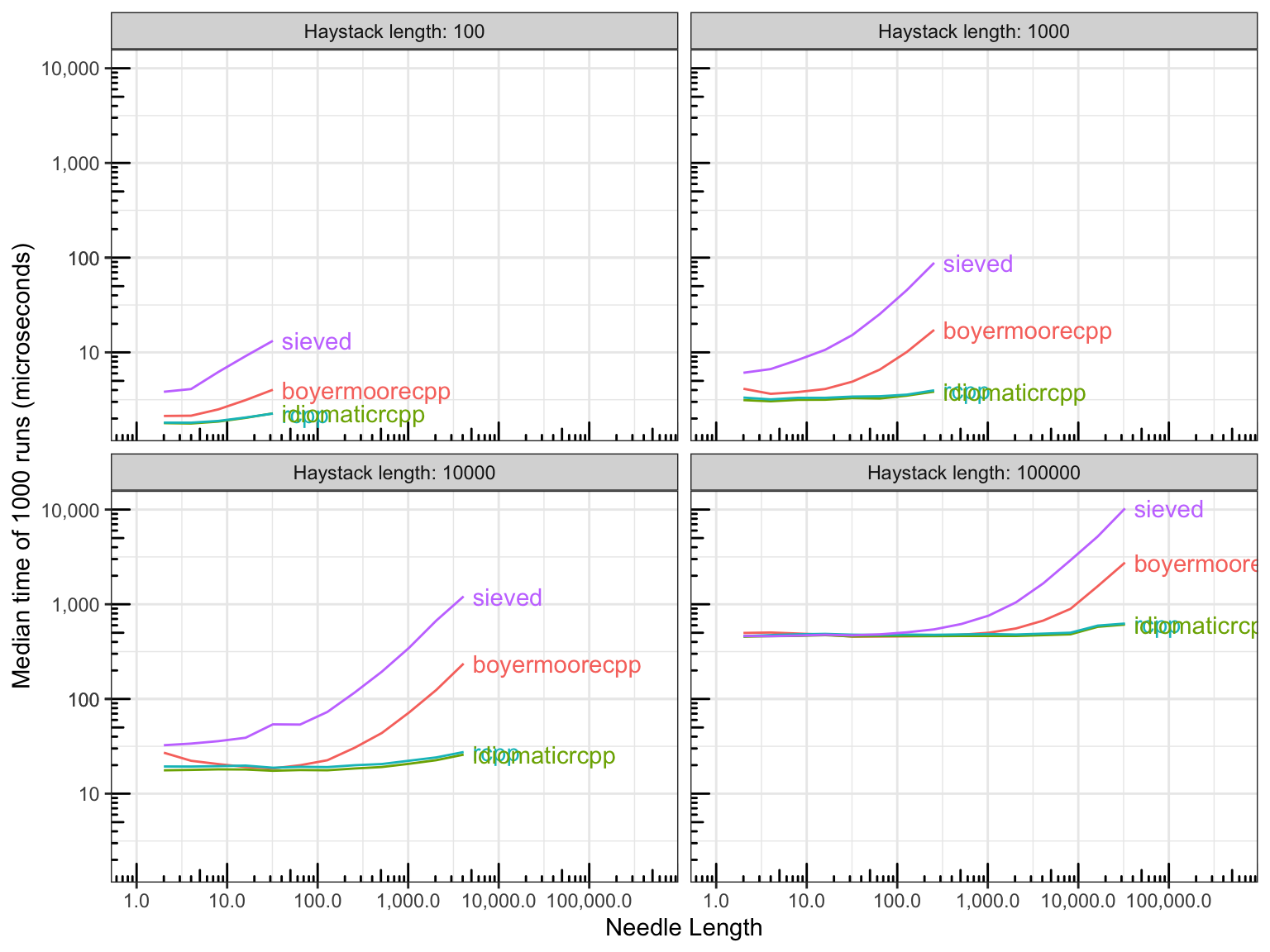# Finding a length n needle in a haystack

## Problem: Find index of a short vector (needle) inside a larger vector (haystack)

What’s a good way to find the location of a `needle` in a `haystack`?

``````haystack <- sample(0:12, size = 2000, replace = TRUE)
needle   <- c(2L, 10L, 8L) ``````
• The `haystack` may be large.
• The `needle` is definitely a vector
• Only the index for the first occurrence of `needle` within `haystack` is needed
• Assuming that there is always at least 1 occurrence

## Solution 1: `for` loop

• Naively test every position in a for loop
• Advantage of this method over a vectorised approach, is that it can terminate early i.e. as soon as a value is found
``````forloop_find <- function(needle, haystack) {
n <- length(needle) - 1L
for (i in seq(haystack)) {
if (identical(haystack[i:(i+n)], needle)) {
return(i)
}
}
}

forloop_find(needle, haystack)``````
``##  931``

## Solution 2: `vapply`

``````vapply_find <- function(needle, haystack) {
n <- length(needle) - 1L
which(vapply(seq(haystack), function(i) {identical(haystack[i:(i+n)], needle)}, FUN.VALUE = logical(1L)))[1L]
}

vapply_find(needle, haystack)``````
``##  931``

## Solution 3: `zoo::rollapply`

``````rollapply_find <- function(needle, haystack) {
which(zoo::rollapply(haystack, length(needle), identical, needle))[1L]
}

rollapply_find(needle, haystack)``````
``##  931``

## Solution 4: stacked `lead()` finding

• for each item in the needle
• shift the haystack and check for the needle element
• stack the shifted haystacks and sum them
• check where `sum == length(needle)`
``````lead_find <- function(needle, haystack) {
v  <- haystack == needle
for (i in seq(2, length(needle))) {
v <- v + (lead(haystack, i-1L) == needle[i])
}

which(v == length(needle))[1L]
}

``##  931``

## Solution 5: `shift`

• `data.table::shift` is a vectorised version of `dplyr::lead()`
• Adapted from user “Jaap” on stackoverflow
``````shift_find <- function(needle, haystack) {

v <- Map('==', shifted_haystack, needle)
v <- Reduce('+', v)

which(v == length(needle))
}

shift_find(needle, haystack)``````
``##  931``

## Solution 5: Rcpp `for` loop

• update 2018-04-04
• Shaved a few % points off the runtime by using the value of `j` instead of an explicit `match` count.
``````Rcpp::cppFunction('int rcpp_find(NumericVector needle, NumericVector haystack) {
int needle_length   = needle.size();
int haystack_length = haystack.size();

for (int i = 0; i < (haystack_length - needle_length); i++ ) {
int j;
for (j = 0; j < needle_length; j++ ) {
if (needle[j] != haystack[i + j]) {
break;
}
}

if (j == needle_length) {
return(i+1);
}
}

return(0);

}')

rcpp_find(needle, haystack)``````
``##  931``

## Solution 6: `pile` find (by dmytro)

• dmytro on twitter offered this one.
``````pile_find <- function(needle, haystack) {
index <- seq_along(haystack)
for (i in seq_along(needle)) {
pile <- haystack[index]
index <- index[pile==needle[i]] + 1L
}
(index-length(needle))
}

pile_find(needle, haystack)``````
``##  931``

## Solution 7: Sieved find (by carroll_jono)

• carroll_jono on twitter offered this one.
• This is the fastest of the pure-R methods.
``````sieved_find <- function(needle, haystack) {
sieved <- which(haystack == needle[1L])
for (i in seq.int(1L, length(needle)-1L)) {
sieved <- sieved[haystack[sieved + i] == needle[i + 1L]]
}
sieved[1L]
}

sieved_find(needle, haystack)``````
``##  931``

## Solution 8: Coerce to string and use grep

• Convert each integer into a string e.g. `c(1, 2, 3)` becomes “001.002.003”
• Current tests are with integers less than 1000, so I’m safe to use `%03i` as a format string.
``````grep_find <- function(needle, haystack) {
expanded_width <- 4  # 3 digits plus a "."
res <- stringr::str_locate_all(paste(sprintf("%03i", haystack), collapse="."), paste(sprintf("%03i", needle), collapse="."))[][,1][]

(res-1)/expanded_width + 1
}

grep_find(needle, haystack)``````
``##  931``

## Solution 9: Idomatic C++ solution hrbrmstr

``````Rcpp::cppFunction('int idiomaticrcpp_find(NumericVector needle, NumericVector haystack) {
NumericVector::iterator it;
it = std::search(haystack.begin(), haystack.end(), needle.begin(), needle.end());
int pos = it - haystack.begin() + 1;
if (pos > haystack.size()) pos = -1;
return(pos);
}')

idiomaticrcpp_find(needle, haystack)``````
``##  931``

## Solution 10: Rcpp boyer-moore jimhester

• Boyer-Moore implementation in Rcpp by jimhester
``````# https://gist.github.com/jimhester/2d02d63d0459d9fdadcf15e08c3afc0a
Rcpp::sourceCpp("cache/2018-04-03-boyer-moore.cpp")
boyermoorecpp_find(needle, haystack)``````
``##  931``

## `needle` is in the middle of the `haystack`

Table 1: `needle` in middle of `haystack`. microbenchmark() timings for 1000 runs - times are in microseconds
expr min lq mean median uq max neval
rollapply_find(needle, haystack) 64622.974 67946.554 78132.17233 80610.9880 83993.7715 261446.362 1000
vapply_find(needle, haystack) 15304.346 16611.288 20492.53295 17070.3485 18881.6200 47565.512 1000
lead_find(needle, haystack) 6057.255 7833.419 14920.53701 8950.0515 18850.4280 199884.346 1000
shift_find(needle, haystack) 2809.894 3108.799 6250.15134 3670.6565 4361.3965 183883.048 1000
forloop_find(needle, haystack) 4768.181 5269.440 6790.98958 5457.1060 5717.7130 29957.481 1000
grep_find(needle, haystack) 3844.324 4002.460 4402.40560 4065.0250 4173.2350 184611.679 1000
pile_find(needle, haystack) 86.056 97.890 289.28966 102.9325 109.6500 21766.544 1000
sieved_find(needle, haystack) 41.337 53.283 75.26908 57.4095 63.0490 7256.632 1000
rcpp_find(needle, haystack) 16.063 22.517 38.00685 27.7005 31.9475 5173.280 1000
idiomaticrcpp_find(needle, haystack) 12.401 20.561 98.40539 25.3500 29.7495 20635.513 1000
boyermoorecpp_find(needle, haystack) 13.654 23.306 86.19733 28.1015 32.6330 23749.599 1000## `needle` is very close to beginning of `haystack`

The `for loop` and `rcpp` are the only methods to benefit from a `needle` that is thought to be at the beginning of the `haystack` i.e. they can both return early as soon as they find match.

When the needle is close to the beginning of the haystack, then the searching time is very short, and the `for loop` is quite competitive with custom `Rcpp` code.

Table 2: `needle` near start of `haystack`. microbenchmark() timings for 1000 runs - times are in microseconds
expr min lq mean median uq max neval
pile_find(needle, haystack) 71.184 126.9605 177.69453 138.3465 163.8710 13695.14 1000
sieved_find(needle, haystack) 46.268 69.6190 83.41948 76.2080 89.6610 223.01 1000
forloop_find(needle, haystack) 23.804 30.7580 53.60288 34.7525 45.3105 11729.11 1000
rcpp_find(needle, haystack) 14.483 47.9385 72.01494 53.3860 62.0890 12677.34 1000
boyermoorecpp_find(needle, haystack) 16.315 51.0210 97.70492 56.7190 67.5945 13618.62 1000## Conclusion

Summary

• `Rcpp` is the fastest way. No real difference between my C-style of C++ and idiomatic C++
• Boyer-Moore in Rcpp no faster than a vanilla solution for current benchmark sizes
• For a pure `R` solution, the `sieve_find()` method is fastest and very competitive with `Rcpp`